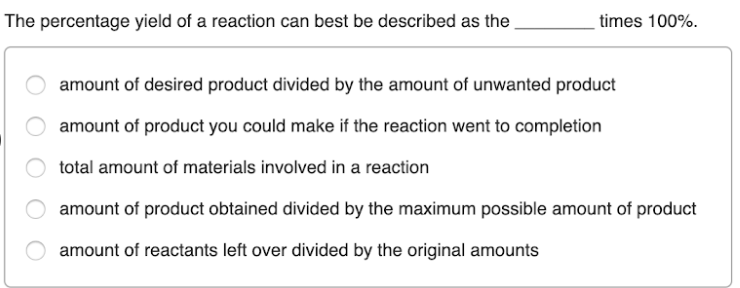# The percentage yield of a reaction can best be described as the _____ times 100%. a. amount of desired product divided by the amount of unwanted product b. amount of product you could make if the reaction went to completion c. total amount of materials involved in a reaction d. amount of product obtained divided by the maximum possible amount of product e. amount of reactants left over divided by the original amounts